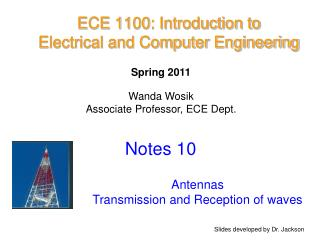DownloadDownload PresentationECE 1100: Introduction to Electrical and Computer Engineering

# ECE 1100: Introduction to Electrical and Computer Engineering

Télécharger la présentation## ECE 1100: Introduction to Electrical and Computer Engineering

- - - - - - - - - - - - - - - - - - - - - - - - - - - E N D - - - - - - - - - - - - - - - - - - - - - - - - - - -
##### Presentation Transcript

1. ECE 1100: Introduction toElectrical and Computer Engineering Spring 2011 Wanda Wosik Associate Professor, ECE Dept. Notes 10 Antennas Transmission and Reception of waves Slides developed by Dr. Jackson

2. Antenna An antenna is used to transmit or receive an electromagnetic signal. A “monopole” antenna is a wire antenna that uses ground as the other terminal.

3. Examples of Monopole Antennas Monopoles on vehicle Cell phone antenna AM tower

4. Transmitting Antenna: Monopole Monopoles are often used for vertical polarization (e.g., AM) The current on a monopole is given by: where i(x,t) h x “monopole antenna”

5. Transmitting Antenna (cont.) For a good antenna, h  / 4 This will maximize the current at the base of the antenna I(0). i(x,t) This follows from: h x The sin term is maximum when “monopole antenna” k

6. base of antenna I(x) top of antenna x h =  / 4 Transmitting Antenna (cont.) i(x,t) h x “monopole antenna”

7. Transmitting Antenna (cont.) Example Pick f= 1,270 kHz = 1.270 MHz (KFCC)  = c / f = 3.0 108 / (1.27 106) = 236.06 meters h So, h 59.0 [m] “monopole antenna”

8. Receive Antenna: Monopole For a good receive antenna, h  / 4 Ex h (The best height for transmitting is also the best height for receiving.) + v(t) - It may be difficult to make the receive antenna this tall – in this case the taller the better! earth “monopole antenna”

9. h h feed (to receiver) L Dipole Antenna A “dipole antenna” is often used for horizontal polarization (as in FM and TV) h  / 4 Example: Pick f = 99.1 MHz (Sunny 99.1 FM)  = c/f = 3.03 m L = 2h  / 2 L= 1.51m

10. Transmission and Reception of Waves

11. Signal Modulation http://hyperphysics.phy-astr.gsu.edu/hbase/hframe.html

12. Amplitude modulation Frequency modulation http://hyperphysics.phy-astr.gsu.edu/hbase/hframe.html

13. Tuning for Signal Detection http://hyperphysics.phy-astr.gsu.edu/hbase/hframe.html

14. Power In Electromagnetic Wave x Ex [V/m] z power flow Hy [A/m] y “intrinsic impedance of free space”

15. x Ex z power flow Hy y Power In Electromagnetic Wave (cont.)

16. Example The AM radio station KFCC (1270 KHz) transmits 50 [kW] of power equally in all directions above the earth (hemisphere pattern). • a) What is the power density 30 [km] away? • What is the magnitude of the electric field 30 [km] away? • Describe mathematically the electric field that an observer would see at this distance. 30 [km] observer

17. Example (cont.) 30 [km] observer

18. Example (cont.) Also, please note that we do not have the amplitude value A. This can be calculated from power: So or

19. Example (cont.) x From previous calculation: Hence we have 30 [km]

20. Ex Hy Receive Voltage Receive antenna wire is in x direction z = zR z h + v(t) - earth Please the units: voltage n[V], electric field E[V/m]

21. Ex Hy Receive Voltage (cont.) h For a monopole antenna: + v(t) - earth z = zR

22. Example Assume that the incident electric field for the AM radio station KFCC has a magnitude of Calculate the received voltage if the vertical antenna wire is 3 meters high. so

23. Example (cont.) (from previous calculation of  and k) So we have: This is the received voltage at the terminals of the monopole antenna.